facet_grid() forms a matrix of panels defined by row and column faceting variables. It is most useful when you have two discrete variables, and all combinations of the variables exist in the data. If you have only one variable with many levels, try facet_wrap().

## Usage

facet_grid(
rows = NULL,
cols = NULL,
scales = "fixed",
space = "fixed",
shrink = TRUE,
labeller = "label_value",
as.table = TRUE,
switch = NULL,
drop = TRUE,
margins = FALSE,
facets = deprecated()
)

## Arguments

rows, cols

A set of variables or expressions quoted by vars() and defining faceting groups on the rows or columns dimension. The variables can be named (the names are passed to labeller).

For compatibility with the classic interface, rows can also be a formula with the rows (of the tabular display) on the LHS and the columns (of the tabular display) on the RHS; the dot in the formula is used to indicate there should be no faceting on this dimension (either row or column).

scales

Are scales shared across all facets (the default, "fixed"), or do they vary across rows ("free_x"), columns ("free_y"), or both rows and columns ("free")?

space

If "fixed", the default, all panels have the same size. If "free_y" their height will be proportional to the length of the y scale; if "free_x" their width will be proportional to the length of the x scale; or if "free" both height and width will vary. This setting has no effect unless the appropriate scales also vary.

shrink

If TRUE, will shrink scales to fit output of statistics, not raw data. If FALSE, will be range of raw data before statistical summary.

labeller

A function that takes one data frame of labels and returns a list or data frame of character vectors. Each input column corresponds to one factor. Thus there will be more than one with vars(cyl, am). Each output column gets displayed as one separate line in the strip label. This function should inherit from the "labeller" S3 class for compatibility with labeller(). You can use different labeling functions for different kind of labels, for example use label_parsed() for formatting facet labels. label_value() is used by default, check it for more details and pointers to other options.

as.table

If TRUE, the default, the facets are laid out like a table with highest values at the bottom-right. If FALSE, the facets are laid out like a plot with the highest value at the top-right.

switch

By default, the labels are displayed on the top and right of the plot. If "x", the top labels will be displayed to the bottom. If "y", the right-hand side labels will be displayed to the left. Can also be set to "both".

drop

If TRUE, the default, all factor levels not used in the data will automatically be dropped. If FALSE, all factor levels will be shown, regardless of whether or not they appear in the data.

margins

Either a logical value or a character vector. Margins are additional facets which contain all the data for each of the possible values of the faceting variables. If FALSE, no additional facets are included (the default). If TRUE, margins are included for all faceting variables. If specified as a character vector, it is the names of variables for which margins are to be created.

facetsPlease use rows and cols instead.

## Examples

p <- ggplot(mpg, aes(displ, cty)) + geom_point()

# Use vars() to supply variables from the dataset:
p + facet_grid(rows = vars(drv))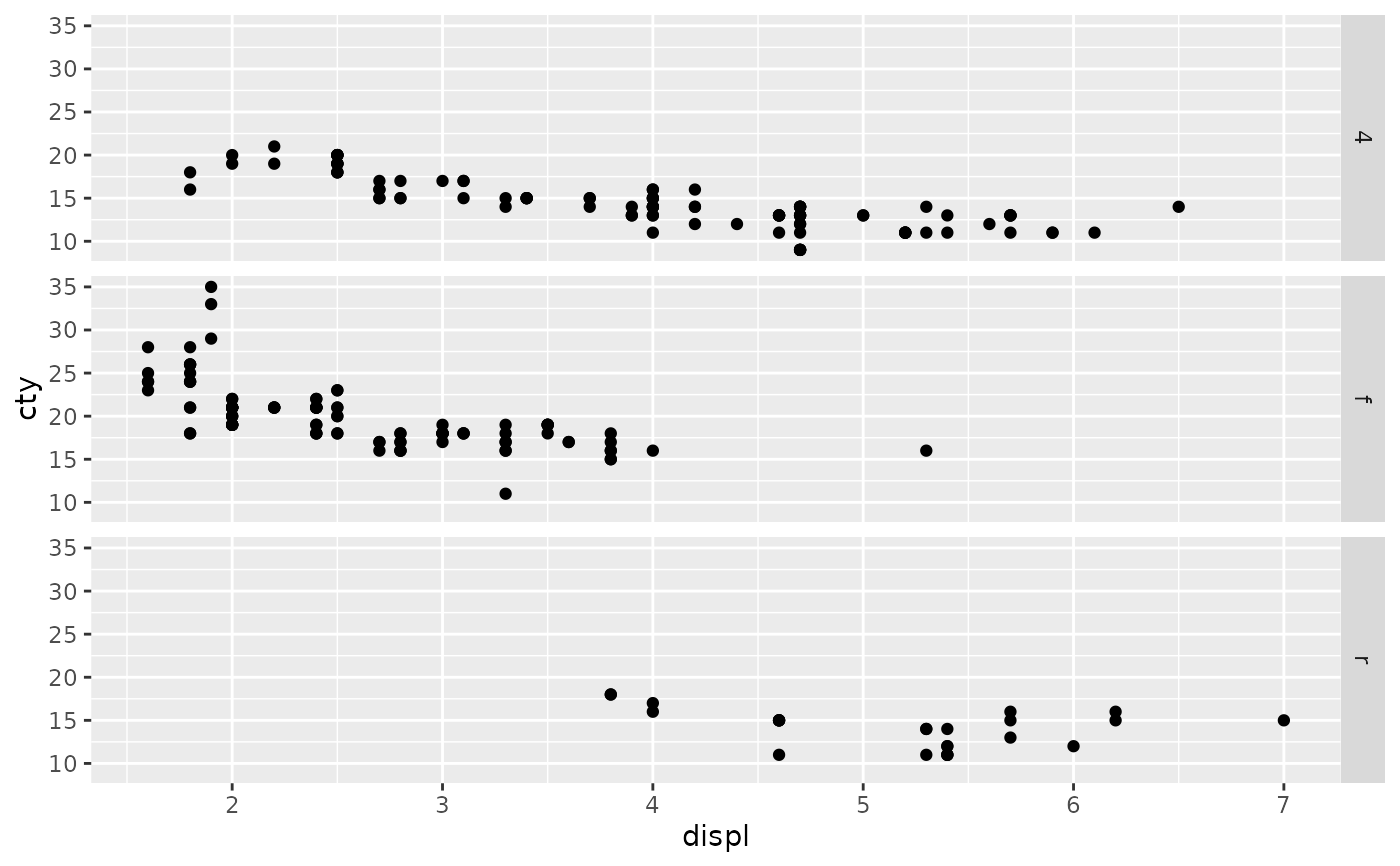p + facet_grid(cols = vars(cyl))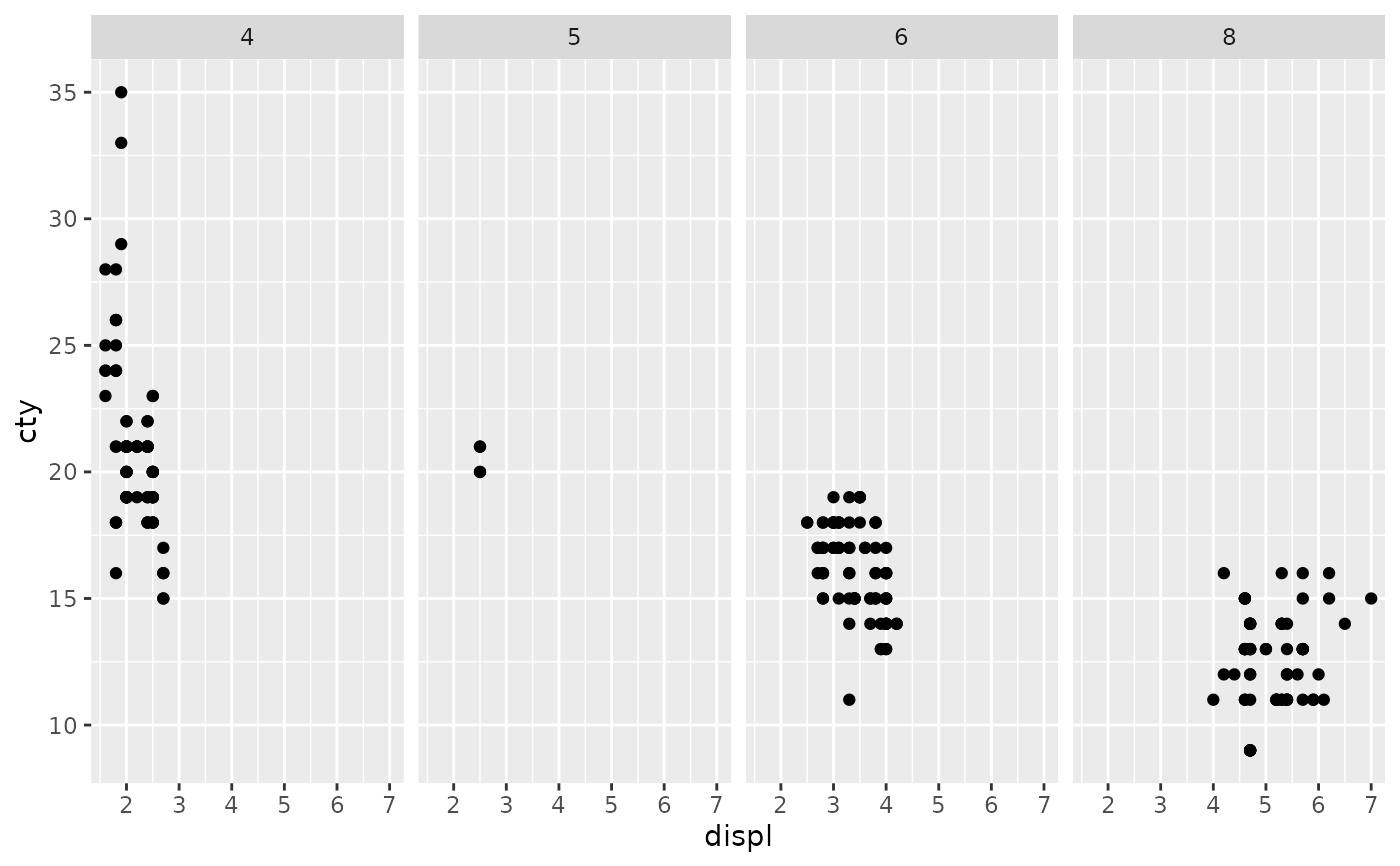p + facet_grid(vars(drv), vars(cyl))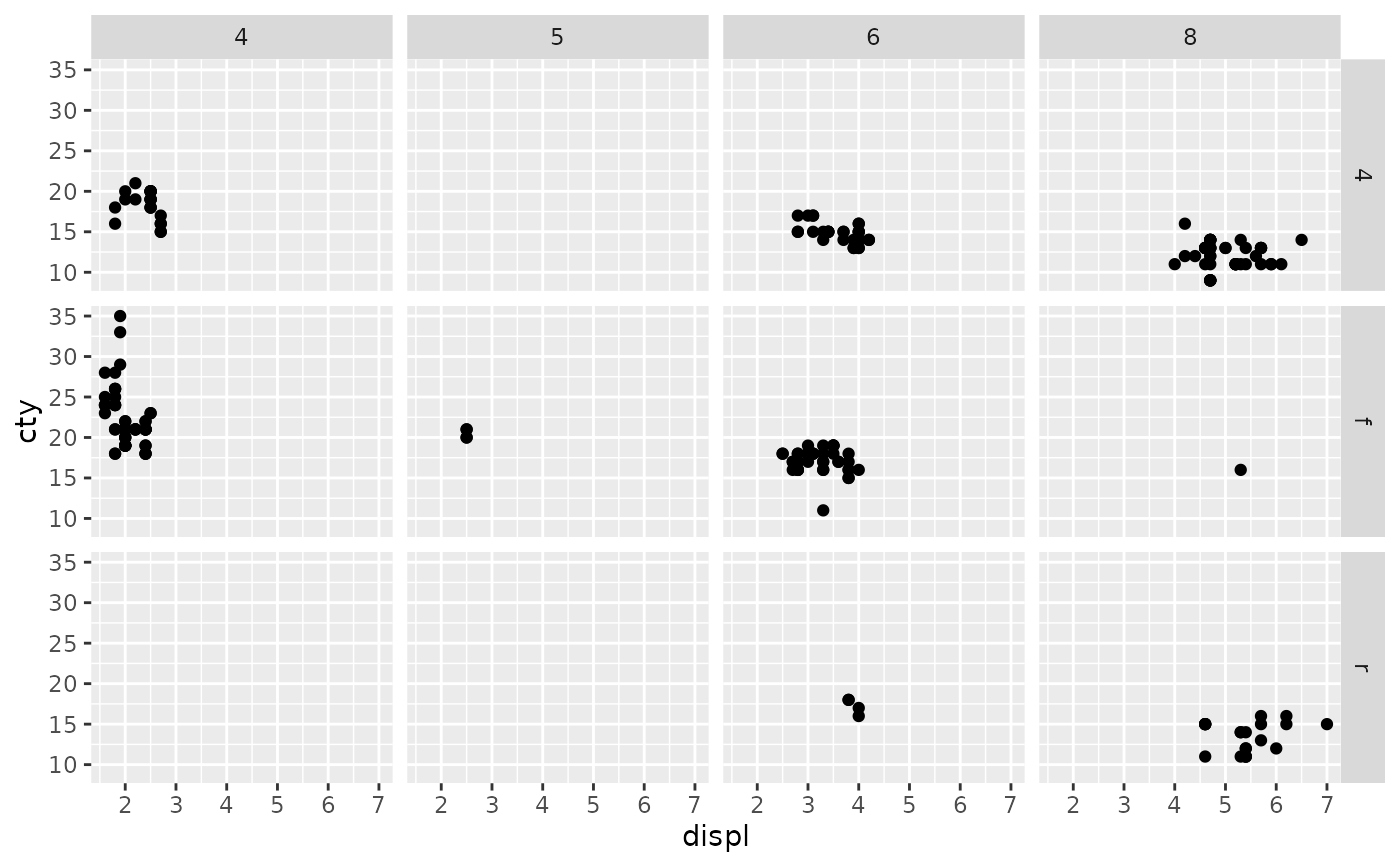# To change plot order of facet grid,
# change the order of variable levels with factor()

# If you combine a facetted dataset with a dataset that lacks those
# faceting variables, the data will be repeated across the missing
# combinations:
df <- data.frame(displ = mean(mpg$displ), cty = mean(mpg$cty))
p +
facet_grid(cols = vars(cyl)) +
geom_point(data = df, colour = "red", size = 2)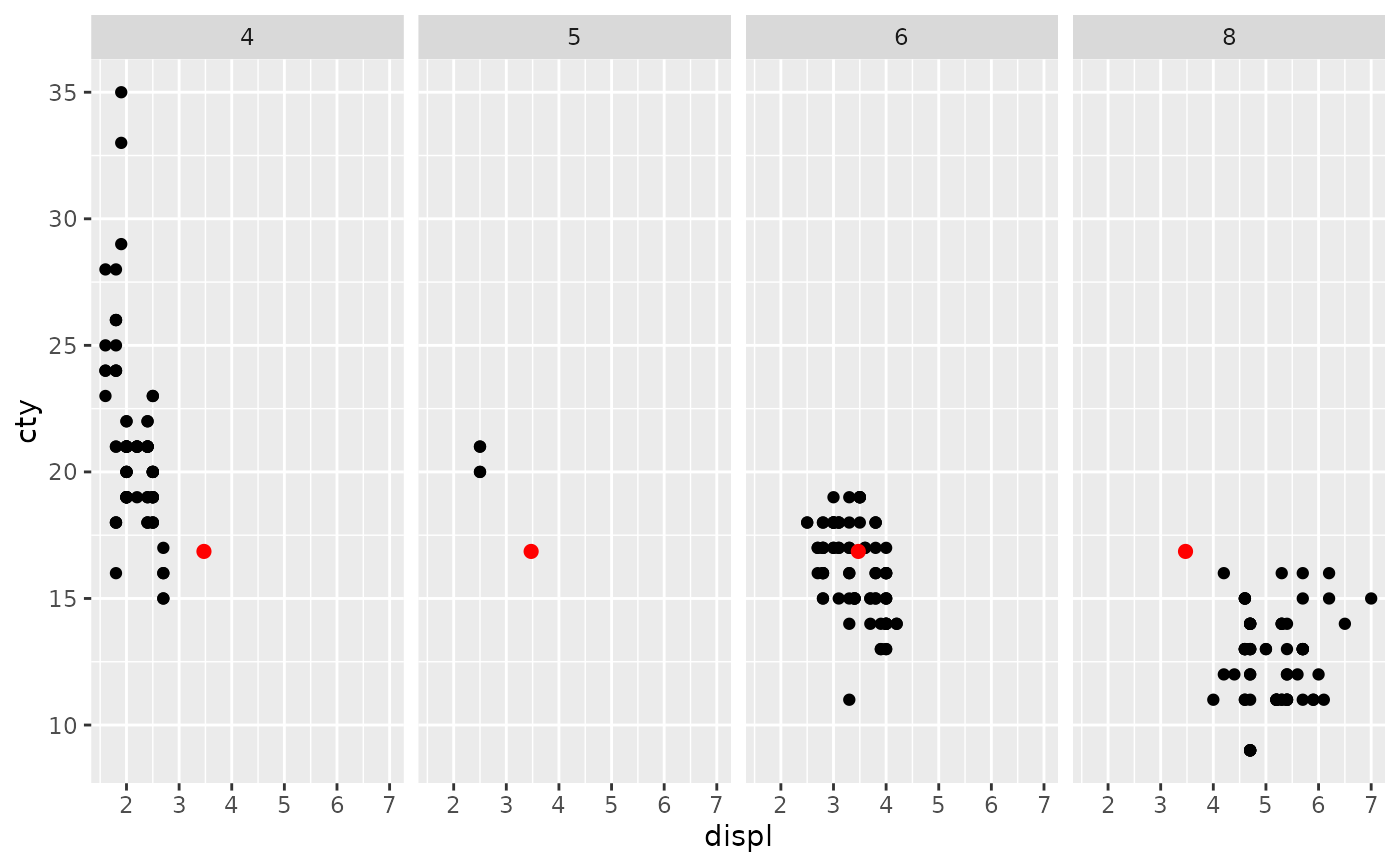# Free scales -------------------------------------------------------
# You can also choose whether the scales should be constant
# across all panels (the default), or whether they should be allowed
# to vary
mt <- ggplot(mtcars, aes(mpg, wt, colour = factor(cyl))) +
geom_point()

mt + facet_grid(vars(cyl), scales = "free")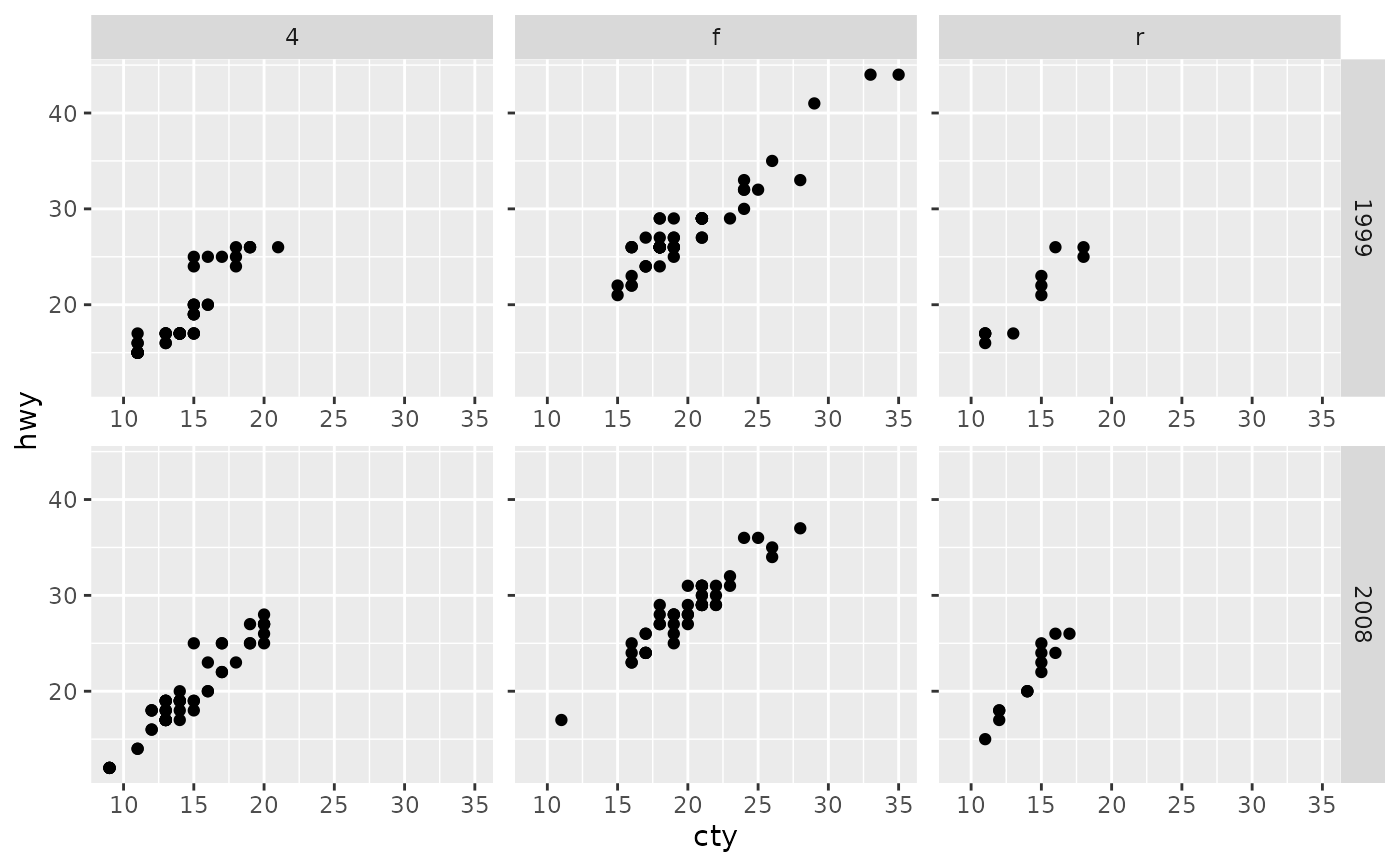# If scales and space are free, then the mapping between position
# and values in the data will be the same across all panels. This
# is particularly useful for categorical axes
ggplot(mpg, aes(drv, model)) +
geom_point() +
facet_grid(manufacturer ~ ., scales = "free", space = "free") +
theme(strip.text.y = element_text(angle = 0))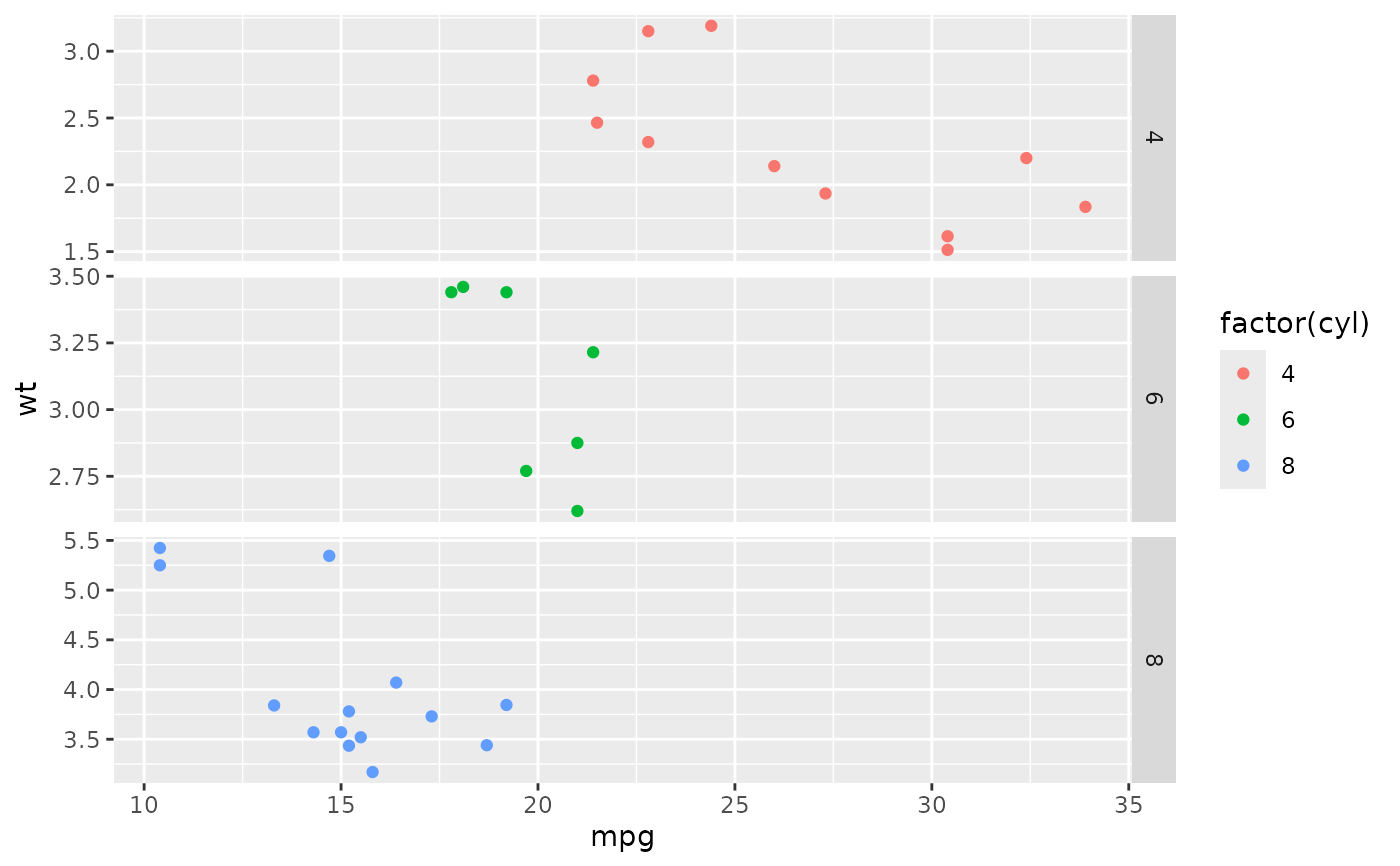# Margins ----------------------------------------------------------
# \donttest{
# Margins can be specified logically (all yes or all no) or for specific
# variables as (character) variable names
mg <- ggplot(mtcars, aes(x = mpg, y = wt)) + geom_point()
mg + facet_grid(vs + am ~ gear, margins = TRUE)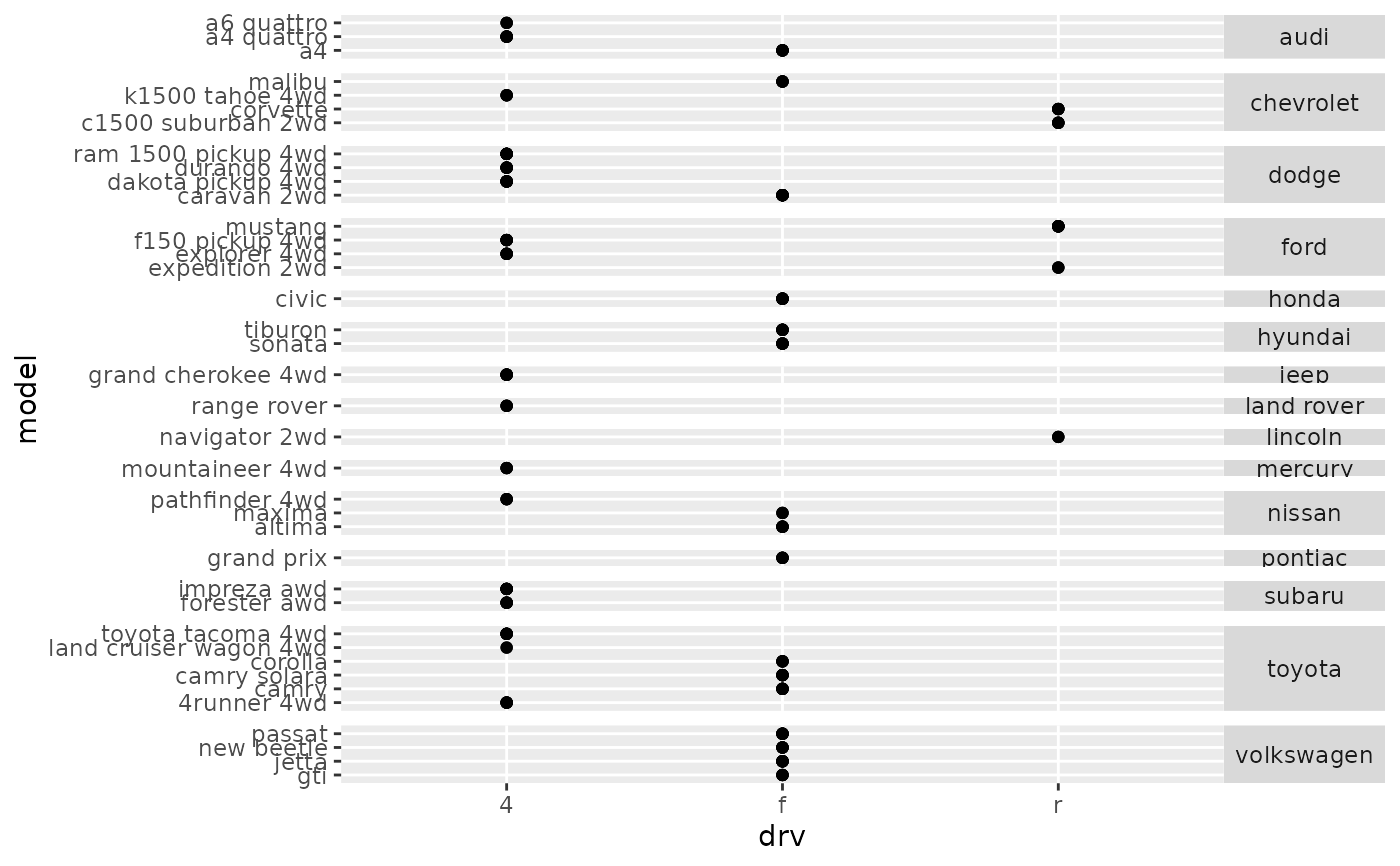mg + facet_grid(vs + am ~ gear, margins = "am")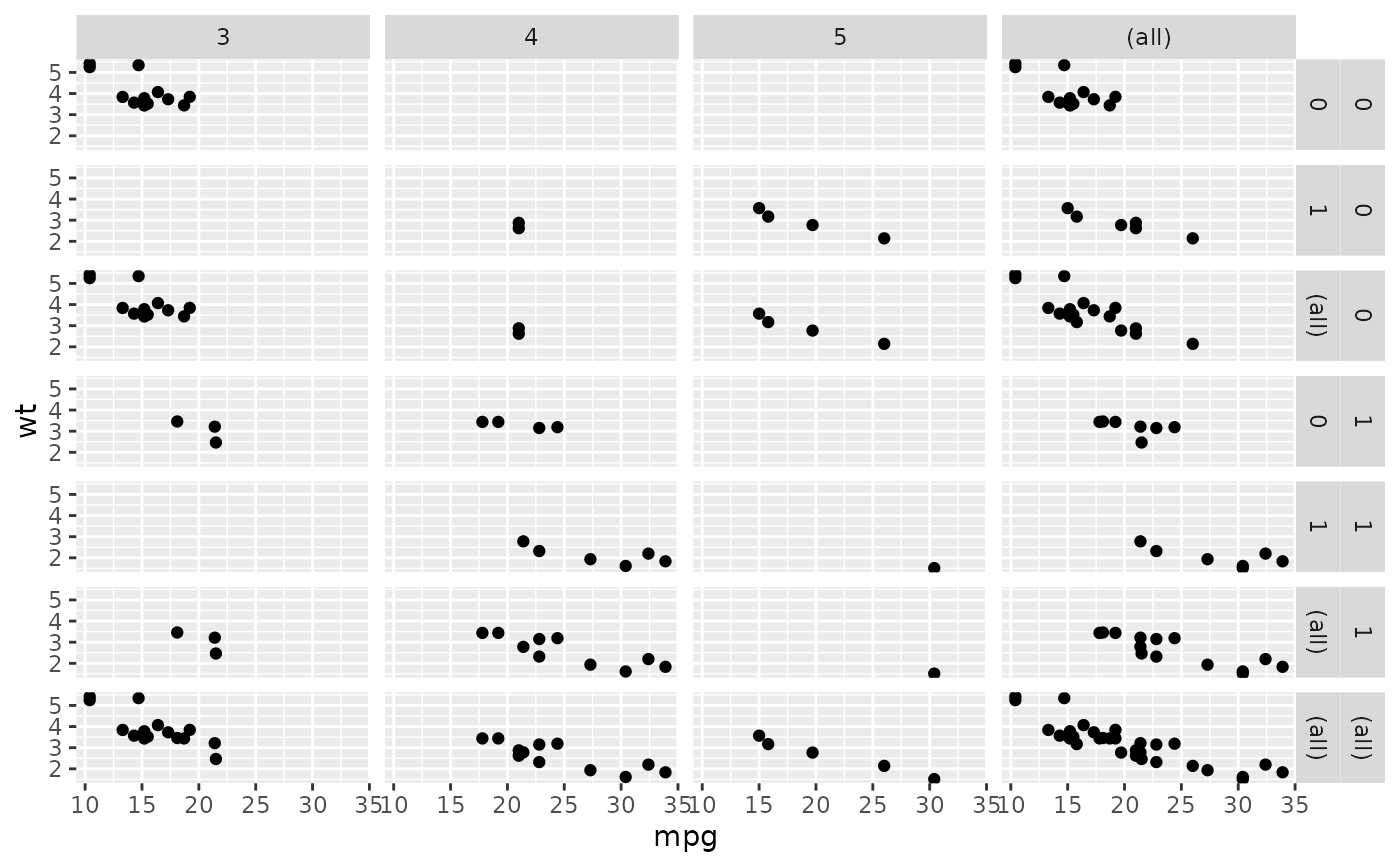# when margins are made over "vs", since the facets for "am" vary
# within the values of "vs", the marginal facet for "vs" is also
# a margin over "am".
mg + facet_grid(vs + am ~ gear, margins = "vs")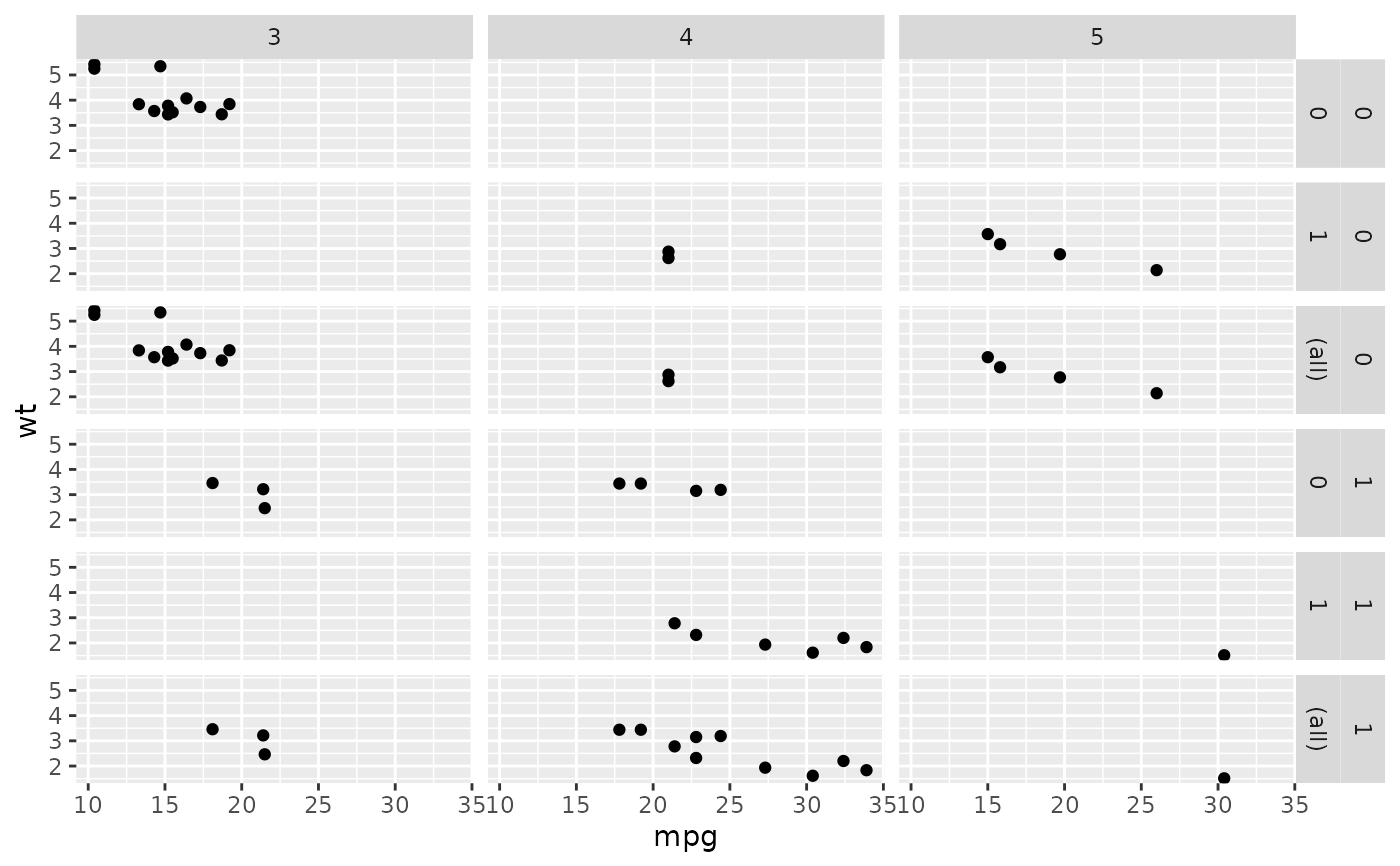# }